SSC  >  SSC CPO Mock Test (GI & Reas) 6

# SSC CPO Mock Test (GI & Reas) 6

Test Description

## 50 Questions MCQ Test SSC CPO & Constable - Mock Tests & Previous Year Papers | SSC CPO Mock Test (GI & Reas) 6

SSC CPO Mock Test (GI & Reas) 6 for SSC 2023 is part of SSC CPO & Constable - Mock Tests & Previous Year Papers preparation. The SSC CPO Mock Test (GI & Reas) 6 questions and answers have been prepared according to the SSC exam syllabus.The SSC CPO Mock Test (GI & Reas) 6 MCQs are made for SSC 2023 Exam. Find important definitions, questions, notes, meanings, examples, exercises, MCQs and online tests for SSC CPO Mock Test (GI & Reas) 6 below.
Solutions of SSC CPO Mock Test (GI & Reas) 6 questions in English are available as part of our SSC CPO & Constable - Mock Tests & Previous Year Papers for SSC & SSC CPO Mock Test (GI & Reas) 6 solutions in Hindi for SSC CPO & Constable - Mock Tests & Previous Year Papers course. Download more important topics, notes, lectures and mock test series for SSC Exam by signing up for free. Attempt SSC CPO Mock Test (GI & Reas) 6 | 50 questions in 30 minutes | Mock test for SSC preparation | Free important questions MCQ to study SSC CPO & Constable - Mock Tests & Previous Year Papers for SSC Exam | Download free PDF with solutions
 1 Crore+ students have signed up on EduRev. Have you?
SSC CPO Mock Test (GI & Reas) 6 - Question 1

### Complete the analogous pair. CBD : FEG :: SRT : ?

SSC CPO Mock Test (GI & Reas) 6 - Question 2

### Re-arrange the given words in alphabetical order and tick the one that comes in the middle.

SSC CPO Mock Test (GI & Reas) 6 - Question 3

### Re-arrange the given words in alphabetical order and tick the one that comes in last.

SSC CPO Mock Test (GI & Reas) 6 - Question 4
Complete the analogous pair.
Rehabilitate : Reinforce :: Restore : ?
SSC CPO Mock Test (GI & Reas) 6 - Question 5
Choose the numeral pair which is different from others .
SSC CPO Mock Test (GI & Reas) 6 - Question 6
Choose the word which is least like the other words in the group .
SSC CPO Mock Test (GI & Reas) 6 - Question 7
Choose the word which is least like the other words in the group.
SSC CPO Mock Test (GI & Reas) 6 - Question 8
Complete the analogous pair.
Sedative : Pain : : Solace : ?
SSC CPO Mock Test (GI & Reas) 6 - Question 9
Complete the analogous pair.
Eye : Man : : Ear : ?
SSC CPO Mock Test (GI & Reas) 6 - Question 10
Complete the analogous pair.
Car : Garage : : Aeroplane : ?
SSC CPO Mock Test (GI & Reas) 6 - Question 11

I am facing East. I turn 100º in the clockwise direction and the 145º in anticlockwise direction. Which direction am I facing now ?

SSC CPO Mock Test (GI & Reas) 6 - Question 12

If the position of the first letter of English alphabet is interchanged with the position of the fourteenth letter, second letter with the fifteenth letter in such a way that M is interchanged with Z, then which of the following letters will be 9th to the right of 17th letter from the right?

SSC CPO Mock Test (GI & Reas) 6 - Question 13

Ravi starting from a fixed point goes 15 km towards North and then after turning to his right he goes 15 km. Then he goes 10, 15 and 15 metres after turning to his left each time. How far is he from his starting point?

SSC CPO Mock Test (GI & Reas) 6 - Question 14
How many squares are there in the following figure?
Detailed Solution for SSC CPO Mock Test (GI & Reas) 6 - Question 14 There are five squares in the upper row of the main figure namely:
AEQM, EGRQ, GISR, IKTS, KBNT

Hence, there are fifteen squares, five in each row of the main figure
Similarly, there are total eight squares - Four in each bigger row of the main figure
And finally there are three squares (AIJD, EKLF and GBCH) from the main figure
Hence, there are a total of twenty six squares in the figure.
SSC CPO Mock Test (GI & Reas) 6 - Question 15
Group the following figures into three classes on the basis of identical properties.
SSC CPO Mock Test (GI & Reas) 6 - Question 16
Find the missing character.
SSC CPO Mock Test (GI & Reas) 6 - Question 17

Choose the odd one out.

SSC CPO Mock Test (GI & Reas) 6 - Question 18

Find the missing character.

Detailed Solution for SSC CPO Mock Test (GI & Reas) 6 - Question 18

In fig. (A), 62 = 36, 82 = 64, 52 = 25, 7 2 = 49. And, 6+8+5+7=26.
In fig. (B), 32 = 9, 52 = 25, 42 = 16, 9 2 = 81. And, 3+5+4+9=21.
In fig. (C), 52 = 25, 122 = 144, 62 = 36, 82 = 64.
So, missing number = 5+12+6+8=31.

SSC CPO Mock Test (GI & Reas) 6 - Question 19
Find the missing character.
Detailed Solution for SSC CPO Mock Test (GI & Reas) 6 - Question 19 In fig. (A), $5�4=20,\phantom{\rule{0.5em}{0ex}}5+4=9.$
In fig. (B), $3�8=24,\phantom{\rule{0.5em}{0ex}}3+8=11.$
$\therefore$In fig. (C), missing number = $9�4=36.$
SSC CPO Mock Test (GI & Reas) 6 - Question 20

Choose the odd one out.

SSC CPO Mock Test (GI & Reas) 6 - Question 21

In the case of how many letters in the word CLIENT, their serial order in the word will not differ than their serial order in the arrangement when the letters of the word are arranged alphabetically?

Detailed Solution for SSC CPO Mock Test (GI & Reas) 6 - Question 21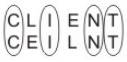4 alphabets would not change their position when arranged alphabetically

SSC CPO Mock Test (GI & Reas) 6 - Question 22

In a certain code NAMES is written as TFNBO. How is CRANE written in that code?

Detailed Solution for SSC CPO Mock Test (GI & Reas) 6 - Question 22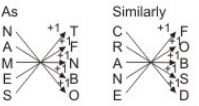SSC CPO Mock Test (GI & Reas) 6 - Question 23

If '√' means 'square', '××' means '+', '-' means '××', '+' means ÷, then
√20 + 25 x 5 − 3 = ?

Detailed Solution for SSC CPO Mock Test (GI & Reas) 6 - Question 23

(20)2 ÷ 25 + 5 x 3
= 400 ÷ 25 + 5 x 3 (use BODMAS Rule)
= 16 + 15 = 31

SSC CPO Mock Test (GI & Reas) 6 - Question 24

If "X" stands for add, 'Y' stands for subtract, 'Z' stands for divide and P stands for multiply, then what is the value of (7 P 3) Y6X5

Detailed Solution for SSC CPO Mock Test (GI & Reas) 6 - Question 24

(7 P 3) Y6X5
= (7 × 3) - 6 + 5
= 21 - 6 + 5
= 26 - 6
= 20

SSC CPO Mock Test (GI & Reas) 6 - Question 25
Which of the following diagrams illustrate the relationship among Science, Maths and Biology?
Detailed Solution for SSC CPO Mock Test (GI & Reas) 6 - Question 25 (A) Biology is a branch of Science. Maths is different from both i.e.
SSC CPO Mock Test (GI & Reas) 6 - Question 26
Which of the following combination of figures best represents Young creatures, Colts and Horses?
SSC CPO Mock Test (GI & Reas) 6 - Question 27

select the correct combination of mathematical signs to replace * sign to balance the given equation.
24*3*2*12*3​

SSC CPO Mock Test (GI & Reas) 6 - Question 28

Complete the analogous pair.
11529 : 72135 :: 152943 : ?

SSC CPO Mock Test (GI & Reas) 6 - Question 29

Choose the odd one out.

SSC CPO Mock Test (GI & Reas) 6 - Question 30
Choose the odd one out.
SSC CPO Mock Test (GI & Reas) 6 - Question 31

Choose the odd one out.

Detailed Solution for SSC CPO Mock Test (GI & Reas) 6 - Question 31

1 : 4
1 × 2 + 2 = 4
8 : 18
8 × 2 + 2 = 18
22 : 46
22 × 2 + 2 = 46
50 : 102
50 × 2 + 2 = 102
10 : 24
10 × 2 + 4 = 24

SSC CPO Mock Test (GI & Reas) 6 - Question 32
3, 10, 29, (66), (127), 218
In the above number series TERMS have been put within bracket. Mark your answer as -
SSC CPO Mock Test (GI & Reas) 6 - Question 33
Find out the term in the number series which is wrong.
6, 14, 30, 64, 126
SSC CPO Mock Test (GI & Reas) 6 - Question 34
Choose the missing term out of the given alternatives.
KM5, IP8, GS11, EV14, ?
SSC CPO Mock Test (GI & Reas) 6 - Question 35
Find the wrong number in the series.
7, 9, 16, 25, 41, 68, 107, 173
Detailed Solution for SSC CPO Mock Test (GI & Reas) 6 - Question 35 The series is 7+9=16;
16+9=25
25+16=41;
41+25=66;
66+41=107,.........
SSC CPO Mock Test (GI & Reas) 6 - Question 36

Find the wrong number in the series.
27 -> 12, 12 -> 8, 33-> 14,

Detailed Solution for SSC CPO Mock Test (GI & Reas) 6 - Question 36

12×2+3 ->12
4×2+4 ->4
14×2+5 ->14

SSC CPO Mock Test (GI & Reas) 6 - Question 37
4, 7, (9), 10, 13, 15, (16), 19
In the above number series TERMS have been put within bracket. Mark your answer as -
SSC CPO Mock Test (GI & Reas) 6 - Question 38
Below is given statement followed by two assumptions numbered I and II. You have to consider the statement and the following assumptions and decide which of the assumptions is implicit in the statement :
Statements :
If he is intelligent he will pass the examination.
Assumptions :
I. To pass, he must be intelligent.
II. He will pass the examination.
SSC CPO Mock Test (GI & Reas) 6 - Question 39
Below is two statements followed by three conclusions numbered I, II, and III. You have to consider the statement and the following conclusions and decide which of the conclusions is follows in the statement :
Statement:
a.All bulbs are tables.
b. Some bulbs are pots.
Conclusion:
I. All pots are tables
II. No pot is table.
III. Some pots are tables.
SSC CPO Mock Test (GI & Reas) 6 - Question 40
If 'spoon' is called 'plate', 'plate' is called knife', 'knife' is called 'jug', 'jug' is called 'glass', 'glass' is called 'saucer' and 'saucer' is called 'spoon', by what do we cut fruit?
SSC CPO Mock Test (GI & Reas) 6 - Question 41
If February 1, 1996 is Wednesday, what day is March 3, 1996 ?
Detailed Solution for SSC CPO Mock Test (GI & Reas) 6 - Question 41 1996 is a leap year and so February has 29 days. Now, 1st, 8th, 15th, 22nd and 29th February has 29 days. So, 1st March is Thursday and 3rd March is Saturday.
SSC CPO Mock Test (GI & Reas) 6 - Question 42
Four of the following five are alike in a certain way and so form a group. Which is the one that does not belong to that group?
SSC CPO Mock Test (GI & Reas) 6 - Question 43
From the given alternative words, select the word which can be formed using the letters of the given word.
PRAGMATIC
SSC CPO Mock Test (GI & Reas) 6 - Question 44

In the following questions, select a figure from amongst the four alternatives, which when placed in the blank space of fig. (X) would complete the pattern.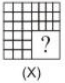SSC CPO Mock Test (GI & Reas) 6 - Question 45

In each question below is given a group of letters followed by four combinations of digits/symbols marked (A), (B), (C) and (D). You have to find which of the combinations correctly represents the groups of letters based on the following coding system and the given conditions and mark the letter of that combination as the answer. If none of the four combinations correctly represents the group of letters, mark (E) i.e. 'None of these' as the answer.
Letter :
A M P J E I R T W B U K Y Z H
Digit/Symbol Code:
1 9 2 @ 3 © 4 8 7 \$ 6 % 5 * δ
Conditions :
(i) If the first letter is consonant and the last letter is a vowel, their codes are to be interchanged.
(ii) If the first letter is vowel and the last letter is consonant, both are to be coded as the code for the vowel.

UPAMYE

SSC CPO Mock Test (GI & Reas) 6 - Question 46

In each question below is given a group of letters followed by four combinations of digits/symbols marked (A), (B), (C) and (D). You have to find which of the combinations correctly represents the groups of letters based on the following coding system and the given conditions and mark the letter of that combination as the answer. If none of the four combinations correctly represents the group of letters, mark (E) i.e. 'None of these' as the answer.
Letter :
A M P J E I R T W B U K Y Z H
Digit/Symbol Code:
1 9 2 @ 3 © 4 8 7 \$ 6 % 5 * δ
Conditions :
(i) If the first letter is consonant and the last letter is a vowel, their codes are to be interchanged.
(ii) If the first letter is vowel and the last letter is consonant, both are to be coded as the code for the vowel.

JPERTB

SSC CPO Mock Test (GI & Reas) 6 - Question 47

Select the correct mirror-image of the Figure (X) from amongst the given alternatives.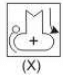SSC CPO Mock Test (GI & Reas) 6 - Question 48

Find out from amongst the four alternatives as to how the pattern would appear when the transparent sheet is folded at the dotted line.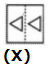SSC CPO Mock Test (GI & Reas) 6 - Question 49

Each of the following question consist of five problem figure marked 1, 2, 3, 4, 5 followed by Answer figure A, B, C, D and E. Select of figure from the answer figure which will continue the same series as given in the problem figure.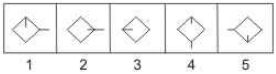SSC CPO Mock Test (GI & Reas) 6 - Question 50

In the questions, figure (X) is embedded in one of following figures. Find out the correct alternative.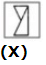## SSC CPO & Constable - Mock Tests & Previous Year Papers

1 docs|63 tests
Information about SSC CPO Mock Test (GI & Reas) 6 Page
In this test you can find the Exam questions for SSC CPO Mock Test (GI & Reas) 6 solved & explained in the simplest way possible. Besides giving Questions and answers for SSC CPO Mock Test (GI & Reas) 6, EduRev gives you an ample number of Online tests for practice

## SSC CPO & Constable - Mock Tests & Previous Year Papers

1 docs|63 tests(Scan QR code)